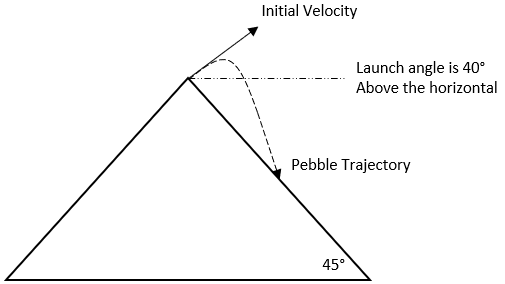## A Pebble on a Mountain

You are at the top of a mountain, and the mountain slopes down below you at a 45° angle below the horizontal. You throw a pebble over the slope, at an angle of 40° above the horizontal. The pebble travels in free-fall for 4 seconds before landing on the slope of the mountain. Its trajectory is shown as the dashed curve in the figure. What was the initial pebble velocity at the moment you threw it?Hint
Motion in the x direction:
$$\Delta x=v_{x}t=(v_{0}cos\alpha )t$$$where $$x$$ is the distance, $$v_0$$ is the initial velocity, $$t$$ is the time, and $$\alpha$$ is the angle. Hint 2 Motion in the y direction: $$\Delta y=v_{y0}t-\frac{1}{2}gt^2=(v_{0}sin\alpha )t-\frac{1}{2}gt^2$$$
where $$x$$ is the distance, $$v_0$$ is the initial velocity, $$t$$ is the time, $$g$$ is the acceleration due to gravity, and $$\alpha$$ is the angle.
First, we need to create some equations to express the motion of direction.

Motion in the x direction:
$$\Delta x=v_{x}t=(v_{0}cos\alpha )t$$$where $$x$$ is the distance, $$v_0$$ is the initial velocity, $$t$$ is the time, and $$\alpha$$ is the angle. Motion in the y direction: $$\Delta y=v_{y0}t-\frac{1}{2}gt^2=(v_{0}sin\alpha )t-\frac{1}{2}gt^2$$$
where $$x$$ is the distance, $$v_0$$ is the initial velocity, $$t$$ is the time, $$g$$ is the acceleration due to gravity, and $$\alpha$$ is the angle. Since the mountain slope is at a 45° angle, $$\Delta x=-\Delta y$$ . So,
$$(v_{0}cos\alpha )t=-(v_{0}sin\alpha)t+\frac{1}{2}gt^2$$$$$v_{0}t(cos\alpha +sin\alpha)=\frac{1}{2}gt^2$$$
$$v_{0}=\frac{(0.5)gt}{(cos\alpha +sin\alpha)}=\frac{(0.5)(9.8m/s^2)(4s)}{cos(40)+sin(40)}$$$$$=\frac{19.6m/s}{1.4}=14 \: m/s$$$
14 m/s